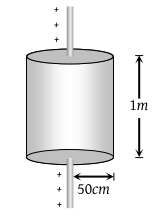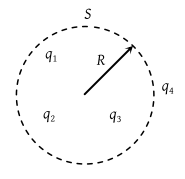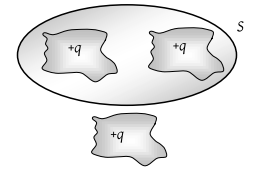Total electric flux coming out of a unit positive charge put in air is

(1) ${\epsilon }_{0}$

(2) ${\epsilon }_{0}^{-1}$

(3) ${\left(4p{\epsilon }_{0}\right)}^{-1}$

(4) $4\pi {\epsilon }_{0}$

Concept Questions :-

Gauss law
High Yielding Test Series + Question Bank - NEET 2020

Difficulty Level:

A cube of side l is placed in a uniform field E, where $E=E\stackrel{^}{i}$. The net electric flux through the cube is

(1) Zero

(2) l2E

(3) 4l2E

(4) 6l2E

Concept Questions :-

Gauss law
High Yielding Test Series + Question Bank - NEET 2020

Difficulty Level:

Eight dipoles of charges of magnitude e are placed inside a cube. The total electric flux coming out of the cube will be

(1) $\frac{8e}{{\epsilon }_{0}}$

(2) $\frac{16e}{{\epsilon }_{0}}$

(3) $\frac{e}{{\epsilon }_{0}}$

(4) Zero

Concept Questions :-

Electric dipole
High Yielding Test Series + Question Bank - NEET 2020

Difficulty Level:

A charge q is placed at the centre of the open end of the cylindrical vessel. The flux of the electric field through the surface of the vessel is

(1) Zero

(2) $\frac{q}{{\epsilon }_{0}}$

(3) $\frac{q}{2{\epsilon }_{0}}$

(4) $\frac{2q}{{\epsilon }_{0}}$

Concept Questions :-

Gauss law
High Yielding Test Series + Question Bank - NEET 2020

Difficulty Level:

According to Gauss’ Theorem, electric field of an infinitely long straight wire is proportional to

(1) r

(2) $\frac{1}{{r}^{2}}$

(3) $\frac{1}{{r}^{3}}$

(4) $\frac{1}{r}$

Concept Questions :-

Gauss law
High Yielding Test Series + Question Bank - NEET 2020

Difficulty Level:

Electric charge is uniformly distributed along a long straight wire of radius 1mm. The charge per cm length of the wire is Q coulomb. Another cylindrical surface of radius 50 cm and length 1m symmetrically encloses the wire as shown in the figure. The total electric flux passing through the cylindrical surface is(1) $\frac{Q}{{\epsilon }_{0}}$

(2) $\frac{100Q}{{\epsilon }_{0}}$

(3) $\frac{10Q}{\left(\pi {\epsilon }_{0}\right)}$

(4) $\frac{100Q}{\left(\pi {\epsilon }_{0}\right)}$

Concept Questions :-

Gauss law
High Yielding Test Series + Question Bank - NEET 2020

Difficulty Level:

The S.I. unit of electric flux is

(1) Weber

(2) Newton per coulomb

(3) Volt × metre

(4) Joule per coulomb

Concept Questions :-

Gauss law
High Yielding Test Series + Question Bank - NEET 2020

Difficulty Level:

q1, q2, q3 and q4 are point charges located at points as shown in the figure and S is a spherical Gaussian surface of radius R. Which of the following is true according to the Gauss’s law ?1. ${\oint }_{s}\left({\stackrel{\to }{E}}_{1}+{\stackrel{\to }{E}}_{2}+{\stackrel{\to }{E}}_{3}\right).d\stackrel{\to }{A}=\frac{{q}_{1}+{q}_{2}+{q}_{3}}{2{\epsilon }_{0}}$

2. ${\oint }_{s}\left({\stackrel{\to }{E}}_{1}+{\stackrel{\to }{E}}_{2}+{\stackrel{\to }{E}}_{3}\right).d\stackrel{\to }{A}=\frac{\left({q}_{1}+{q}_{2}+{q}_{3}\right)}{{\epsilon }_{0}}$

3. ${\oint }_{s}\left({\stackrel{\to }{E}}_{1}+{\stackrel{\to }{E}}_{2}+{\stackrel{\to }{E}}_{3}\right).d\stackrel{\to }{A}=\frac{\left({q}_{1}+{q}_{2}+{q}_{3}+{q}_{4}\right)}{{\epsilon }_{0}}$

4. None of the above

Concept Questions :-

Gauss law
High Yielding Test Series + Question Bank - NEET 2020

Difficulty Level:

If the electric flux entering and leaving an enclosed surface respectively is ${\phi }_{1}$ and ${\phi }_{2}$ the electric charge inside the surface will be

(1) $\left({\phi }_{1}+{\phi }_{2}\right){\epsilon }_{0}$

(2) $\left({\phi }_{2}-{\phi }_{1}\right){\epsilon }_{0}$

(3) $\left({\phi }_{1}+{\phi }_{2}\right)/{\epsilon }_{0}$

(4) $\left({\phi }_{2}-{\phi }_{1}\right)/{\epsilon }_{0}$

Concept Questions :-

Gauss law
High Yielding Test Series + Question Bank - NEET 2020

Difficulty Level:

Shown below is a distribution of charges. The flux of electric field due to these charges through the surface S is(1) $3q/{\epsilon }_{0}$

(2) $2q/{\epsilon }_{0}$

(3) $q/{\epsilon }_{0}$

(4) Zero

Concept Questions :-

Gauss law